# Solids Liquids Energy Heat Intermolecular Forces Inter between

• Slides: 28Solids, Liquids, Energy & HeatIntermolecular Forces “Inter” => between “molecular” => molecules Intermolecular forces apply only to covalent bondsIntermolecular Forces vs. Intramolecular Forces • Intramolecular forces are within one molecule • a. k. a. Bonding • Stronger than intermolecular forces • Types: • Ionic Bonding • Covalent Bonding Intermolecular Forces • Intermolecular forces are between neighboring molecules • Weaker than intramolecular forces • Types: • London dispersion forces • dipole-dipole forces • hydrogen bonding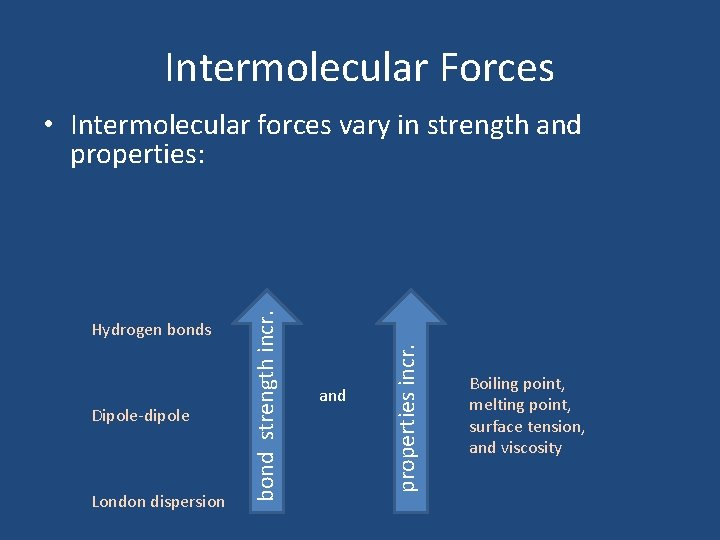Intermolecular Forces Dipole-dipole London dispersion and properties incr. Hydrogen bonds bond strength incr. • Intermolecular forces vary in strength and properties: Boiling point, melting point, surface tension, and viscosityLondon Dispersion Forces (van der Waals Forces) • Very weak forces • Occurs when an electron cloud temporarily gets distorted and forms a temporary positive and negative charge. • The ONLY forces of attraction that exist between nonpolar molecules (e. g. Ar, C 8 H 18 )London Dispersion ForcesDipole-Dipole Attraction • Polar molecules that orient themselves so that + and – ends of the dipoles are close to each other.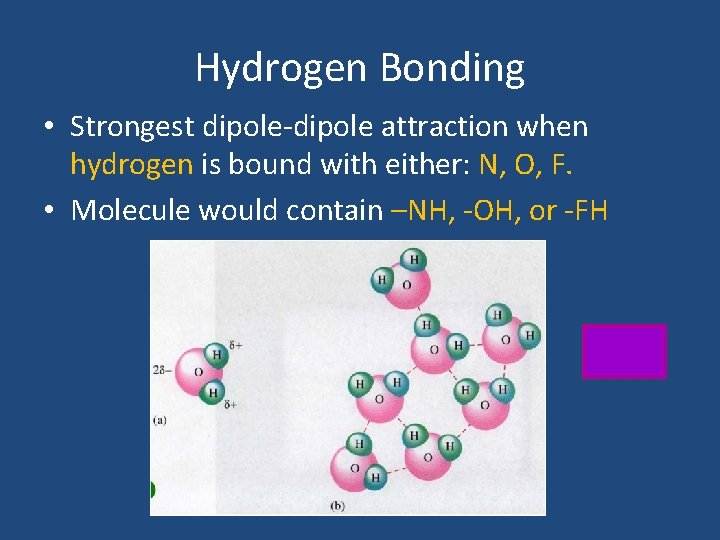Hydrogen Bonding • Strongest dipole-dipole attraction when hydrogen is bound with either: N, O, F. • Molecule would contain –NH, -OH, or -FHHydrogen Bonding in DNA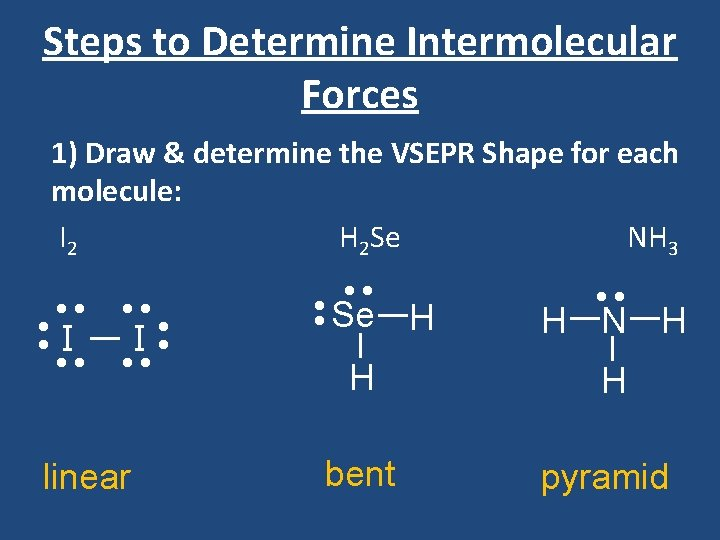Steps to Determine Intermolecular Forces 1) Draw & determine the VSEPR Shape for each molecule: I 2 H 2 Se NH 3 • • • • I I • • • • linear • • • • Se H • • H N H H H bent pyramid2) Determine if each molecule is a polar or nonpolar structure: Options: (a) There are no polar bonds non-polar structure (b) Every polar bond has an opposite (no lone pairs on center) non-polar structure (c) Not every polar bond has an opposite (lone pairs on center) polar structure • • • • • • I 2 • I • • • I • • • non-polar • Se H H 2 Se. H polar H N H H NH 3 polar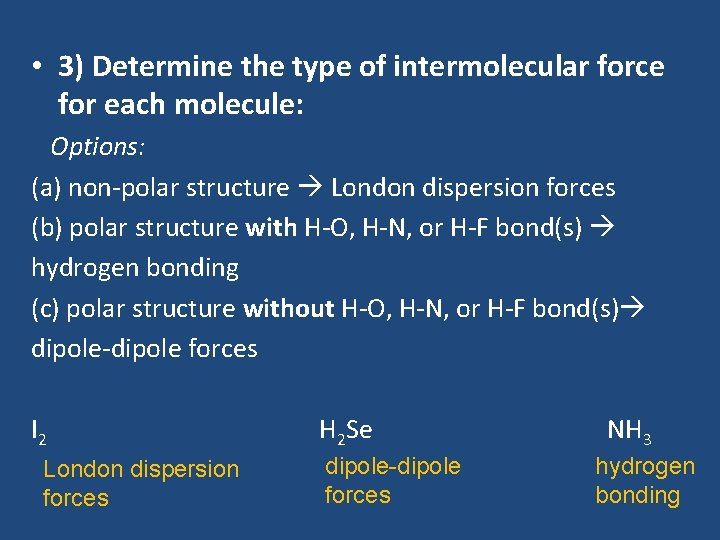• 3) Determine the type of intermolecular force for each molecule: Options: (a) non-polar structure London dispersion forces (b) polar structure with H-O, H-N, or H-F bond(s) hydrogen bonding (c) polar structure without H-O, H-N, or H-F bond(s) dipole-dipole forces I 2 H 2 Se NH 3 London dispersion forces dipole-dipole forces hydrogen bonding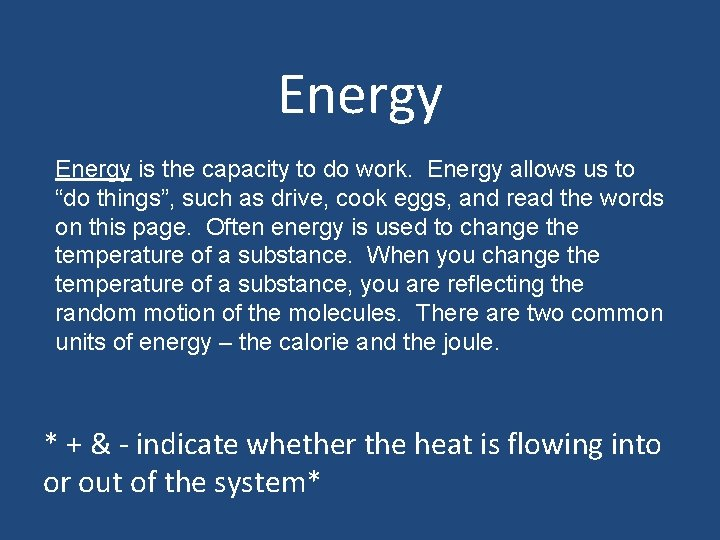Energy is the capacity to do work. Energy allows us to “do things”, such as drive, cook eggs, and read the words on this page. Often energy is used to change the temperature of a substance. When you change the temperature of a substance, you are reflecting the random motion of the molecules. There are two common units of energy – the calorie and the joule. * + & - indicate whether the heat is flowing into or out of the system*A calorie (cal) is defined as the amount of energy required to raise the temperature of one gram of water by 1 C. The joule (J) is the unit of energy in the SI System. 1 calorie = 4. 184 J Example – Express 22. 4 cal of energy in joules. 4. 184 J 22. 4 cal X _________ = 93. 7 J 1 cal 1 kcal = 1000 cal 1 k. J = 1000 JThe amount of energy required to raise the temperature of a substance depends on three things: 1. amount of substance (mass) 2. the amount of the temperature change 3. type or identity of the substance Different substances respond to heat differently – 4. 184 J raises 1 g of water - 1 C BUT 4. 184 J raises gold 32 C Specific Heat Capacity – the amount of energy required to change the temperature of one gram of a substance by 1 C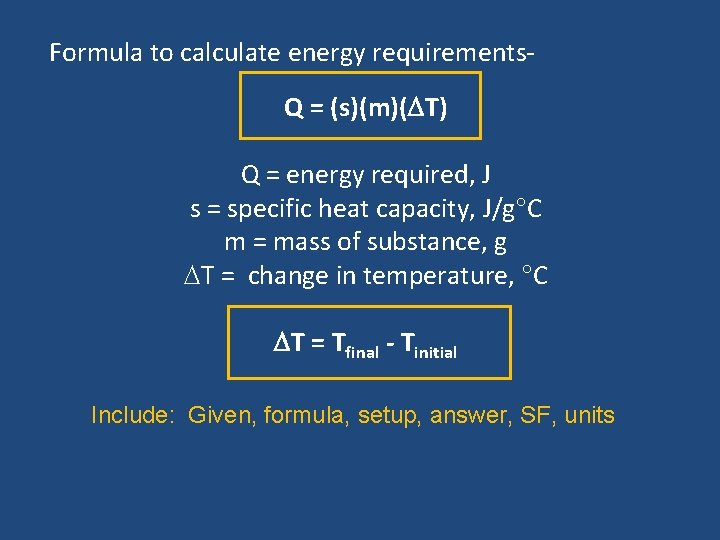Formula to calculate energy requirements. Q = (s)(m)( T) Q = energy required, J s = specific heat capacity, J/g C m = mass of substance, g T = change in temperature, C T = Tfinal - Tinitial Include: Given, formula, setup, answer, SF, units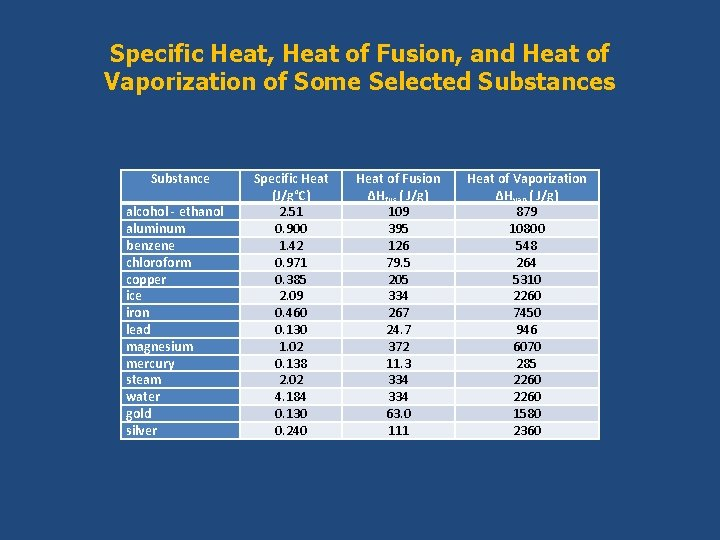Specific Heat, Heat of Fusion, and Heat of Vaporization of Some Selected Substances Substance alcohol - ethanol aluminum benzene chloroform copper ice iron lead magnesium mercury steam water gold silver Specific Heat (J/g°C) 2. 51 0. 900 1. 42 0. 971 0. 385 2. 09 0. 460 0. 130 1. 02 0. 138 2. 02 4. 184 0. 130 0. 240 Heat of Fusion ΔHfus ( J/g) 109 395 126 79. 5 205 334 267 24. 7 372 11. 3 334 63. 0 111 Heat of Vaporization ΔHvap ( J/g) 879 10800 548 264 5310 2260 7450 946 6070 285 2260 1580 2360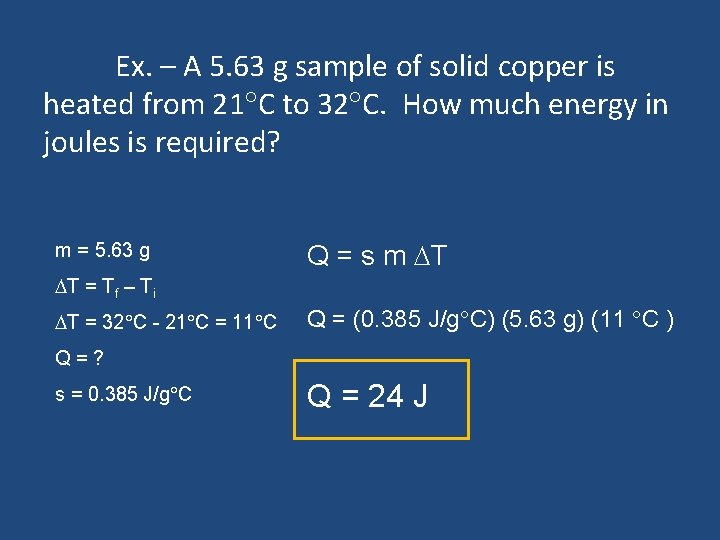Ex. – A 5. 63 g sample of solid copper is heated from 21 C to 32 C. How much energy in joules is required? m = 5. 63 g Q = s m T T = Tf – Ti T = 32 C - 21 C = 11 C Q = (0. 385 J/g C) (5. 63 g) (11 C ) Q = ? s = 0. 385 J/g C Q = 24 JExample – A 2. 8 g sample of pure metal requires 10. 1 J of energy to change its temperature from 21 C to 36 C. What is the metal? m = 2. 8 g Q = 10. 1 J T = Tf – Ti T = 36 C - 21 C = 15 C s = ? s = __Q__ m T Look on chart s = ___10. 1 J___ (2. 8 g)(15 C) s = 0. 24 J/g C Silver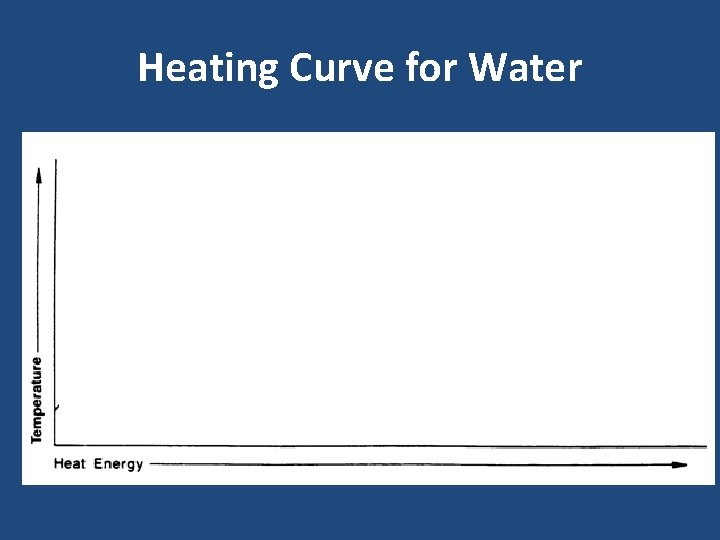Heating Curve for Water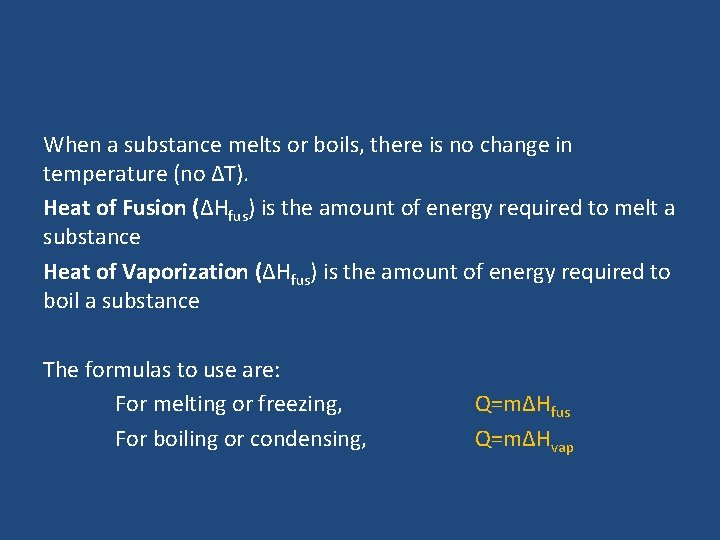When a substance melts or boils, there is no change in temperature (no ∆T). Heat of Fusion (∆Hfus) is the amount of energy required to melt a substance Heat of Vaporization (∆Hfus) is the amount of energy required to boil a substance The formulas to use are: For melting or freezing, Q=m∆Hfus For boiling or condensing, Q=m∆HvapMolar Heat of Fusion and Molar Heat of Vaporization have units of J/mol Heat of Fusion, Heat of Vaporization, and Specific Heat capacity are characteristic properties and can be used to identify a substance. Ex. How much energy is required to melt 8. 93 moles of gold with no change in temperature? q=m∆Hfus g Au 196. 97 m = 8. 93 mol Au x _____ = 1760 g Au q = (1760 g)(63. 0 J/g) 1 mol Au Hfus Au = 63. 0 J/g q = 111000 J Ex. How much energy is absorbed when 5. 34 g of chloroform condenses from a gas? q=m∆Hvap m = 5. 34 g q = (5. 34 g)(264 J/g) Hvap chloroform = 264 J/g q = 1410 J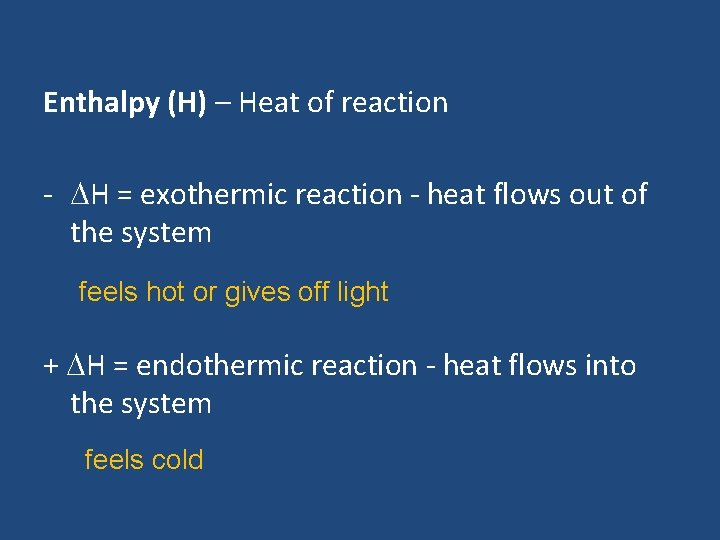Enthalpy (H) – Heat of reaction - H = exothermic reaction - heat flows out of the system feels hot or gives off light + H = endothermic reaction - heat flows into the system feels cold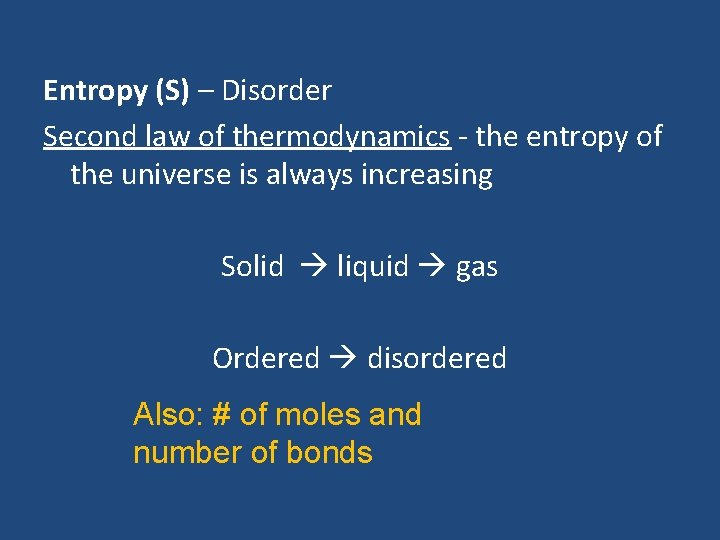Entropy (S) – Disorder Second law of thermodynamics - the entropy of the universe is always increasing Solid liquid gas Ordered disordered Also: # of moles and number of bondsEx: Which of the following has more entropy? a. solid or gaseous phosphorus b. KBr (s) or KBr (aq) c. CH 4 (g) or C 3 H 8 (g)Ex: Does entropy increase or decrease for each of the following reactions? a. (NH 4)2 Cr 2 O 7 (s) Cr 2 O 3 (s) + 4 H 2 O (l) + N 2 (g) INCREASE b. Mg(OH)2 (s) Mg. O (s) + H 2 O (g) INCREASE c. PCl 3 (g) + Cl 2 (g) PCl 5 (g) DECREASEPhase Diagram • Triple point is the temperature and pressure at which all three phases can exist in equilibrium. Above the critical point, molecules are unable to liquefy.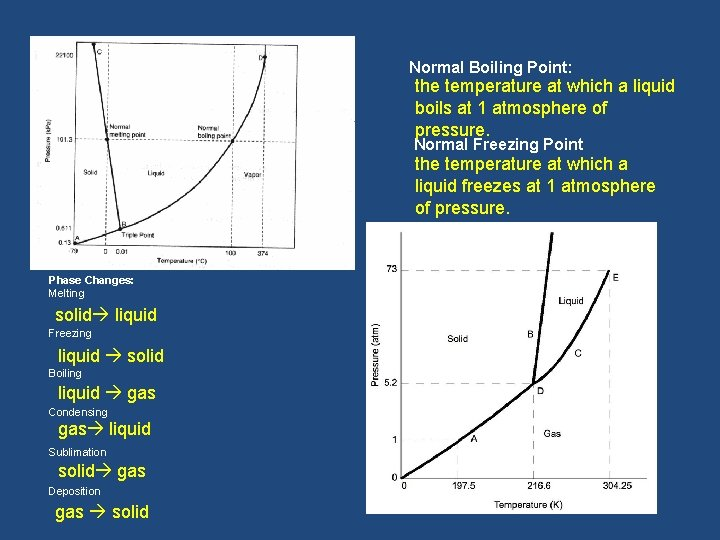Normal Boiling Point: the temperature at which a liquid boils at 1 atmosphere of pressure. Normal Freezing Point the temperature at which a liquid freezes at 1 atmosphere of pressure. Phase Changes: Melting Freezing Boiling Condensing Sublimation Deposition solid liquid gas liquid solid gas solid